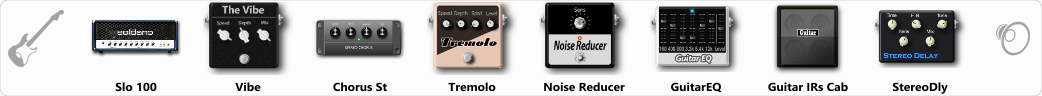Discussion in 'ToneLib-GFX presets' started by Tetragramm1983, Jun 5, 2019.

1. ### Tetragramm1983Active Member

GFX version: 3.0

Optimized for: Guitar Amp

Effects chain:Lead Rhythm or Overlay. Best use with power Octaves!

Effect: "Slo 100" (Amp simulators), active - "yes"
"Gain" = 10
"Bass" = 60
"Middle" = 78
"Treble" = 60
"Presence" = 81
"Master" = 89
"Output" = 50
"Level (dB)" = 10

Effect: "Vibe" (Modulation / Sfx), active - "yes"
"Speed" = 1.9
"Depth" = 38
"Mix" = 10

Effect: "Chorus St" (Modulation / Sfx), active - "yes"
"Speed" = 1.9
"Depth" = 85
"Center" = 10.0
"Mix" = 100

Effect: "Tremolo" (Modulation / Sfx), active - "yes"
"Speed" = 2.9
"Depth" = 47
"Level" = 25

Effect: "Noise Reducer" (Dynamics / Filter), active - "yes"
"Sens" = 100
"Mode" = Hard

Effect: "GuitarEQ" (Dynamics / Filter), active - "yes"
"160 Hz" = -3
"400 Hz" = -8
"800 Hz" = 3
"1.6 kHz" = 3
"3.2 kHz" = -4
"6.4 kHz" = -3
"12 kHz" = -10
"Level (dB)" = 0

Effect: "Guitar IRs Cab" (Cabinets), active - "yes"
"Model" = Orange PPC (4x12")
"Mic Position" = Edge
"Mic Distance" = Near
"Low Cut (Hz)" = 88
"Hi Cut (kHz)" = 8.5
"Mix" = 100
"Level (dB)" = 2

Effect: "StereoDly" (Delay), active - "yes"
"Time" = Note sync, 1/8 Triplet
"Feedback" = 65
"Tone" = 85
"Sens" = 25
"Mix" = 88

Note: This is a ToneLib-GFX preset file, you will need to download and install the ToneLib-GFX software to use the preset.